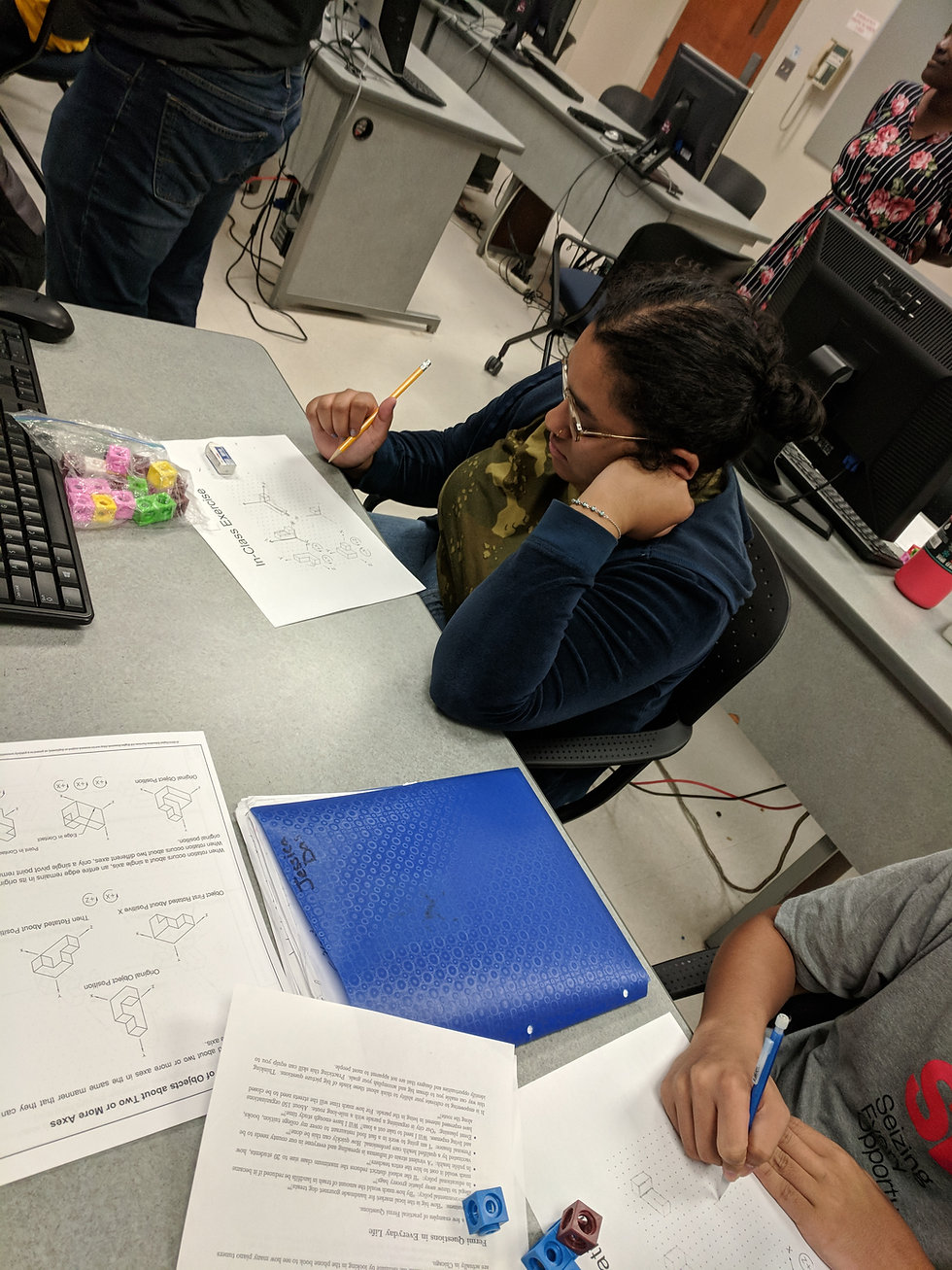Engineering Knowledge: Engineering Sciences## Engineering Performance Matrix

Statics is a fundamental physics concept that focuses on the equilibrium of bodies that are subjected to a force system. It primarily concerns the application of Newton’s laws of motion to analyze loads placed on objects at rest or at a constant velocity. Because these objects are resting or have a constant velocity, the sum of all of the forces applied to the object must be equal to zero. Statics is important to Engineering Literacy, as it is the basis on which engineering professionals analyze physical systems that are void of acceleration. For example, the application of statics enables the analysis of forces applied to physical objects/systems such as trusses, cables, and chains. In addition, statics enables engineering professionals to calculate the magnitudes of the components of forces applied to an object using a series of equations.

## Performance Goal for High School Learners

I can, when appropriate, draw upon the knowledge of statics content, such as (a) determining the resultants of force systems, (b) finding equivalent force systems, (c) conditions of equilibrium for rigid bodies, (d) the analysis of frames/trusses, (e) finding the centroid of an area, and (f) calculating area moments of inertia, to analyze the forces within a static system to solve problems in a manner that is analytical, predictive, repeatable, and practical.

## RESULTANTS OF FORCE SYSTEMS

I can describe resultants of force systems, using appropriate examples.

## EQUIVALENT FORCE SYSTEMS

I can describe equivalent force systems, using appropriate examples.

I can explain the resultant force of a system, drawing free diagrams.

I can explain the conditions for resultants force of equivalent systems, drawing free diagrams.

I can determine resultant problems, using correct mathematical equations and units.

I can assess and apply two equivalent force systems, using correct mathematical equations and units.

## EQUILIBRIUM OFRIGID BODIES

I can describe equilibrium of rigid bodies, using appropriate examples.

I can explain the conditions for equilibrium of a rigid body by drawing free body diagrams.

I can evaluate and assess equilibrium of a rigid-body, using correct mathematical equations and units.

## FRAMES AND TRUSSES

I can describe frames and trusses, using appropriate examples.

I can explain force members of different types of frames and trusses, drawing free body diagrams.

I can determine forces in members of a frame or truss, using correct mathematical equations and units.

## CENTROID OF AREA

I can describe centroid of area, using appropriate examples.

I can explain the centroid of an area on an axis coordinate plane.

I can determine centroid of areas, using the integration method and correct units.

## AREA MOMENTSOF INERTIA

I can describe area moments of inertia, using appropriate examples.

I can explain area moments of inertia in terms of different shapes (e.g. rectangular, circular, triangle, I, T, channel, etc.) on the coordinate plane.

I can determine area moments of inertia, correct mathematical equations and units.